# GcsSloop

Just do IT later.

# 一.Path常用方法表

rXxx方法 rMoveTo, rLineTo, rQuadTo, rCubicTo 不带r的方法是基于原点的坐标系(偏移量)， rXxx方法是基于当前点坐标系(偏移量)

reset不保留内部数据结构，但会保留FillType.
rewind会保留内部的数据结构，但不保留FillType

# 二.Path详解

## Path含义

The Path class encapsulates compound (multiple contour) geometric paths consisting of straight line segments, quadratic curves, and cubic curves. It can be drawn with canvas.drawPath(path, paint), either filled or stroked (based on the paint’s Style), or it can be used for clipping or to draw text on a path.

Path封装了由直线和曲线(二次，三次贝塞尔曲线)构成的几何路径。你能用Canvas中的drawPath来把这条路径画出来(同样支持Paint的不同绘制模式)，也可以用于剪裁画布和根据路径绘制文字。我们有时会用Path来描述一个图像的轮廓，所以也会称为轮廓线(轮廓线仅是Path的一种使用方法，两者并不等价)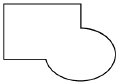封闭路径 首尾相接形成了一个封闭区域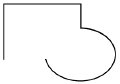开放路径 没有首尾相接形成封闭区域

## Path使用方法详解### 第1组: moveTo、 setLastPoint、 lineTo 和 close

``````Paint mPaint = new Paint();             // 创建画笔
mPaint.setColor(Color.BLACK);           // 画笔颜色 - 黑色
mPaint.setStyle(Paint.Style.STROKE);    // 填充模式 - 描边
mPaint.setStrokeWidth(10);              // 边框宽度 - 10
``````

#### lineTo：

``````public void lineTo (float x, float y)
``````

lineTo很简单，只有一个方法，作用也很容易理解，line嘛，顾名思义就是一条线。

``````canvas.translate(mWidth / 2, mHeight / 2);  // 移动坐标系到屏幕中心(宽高数据在onSizeChanged中获取)

Path path = new Path();                     // 创建Path

path.lineTo(200, 200);                      // lineTo
path.lineTo(200,0);

canvas.drawPath(path, mPaint);              // 绘制Path
``````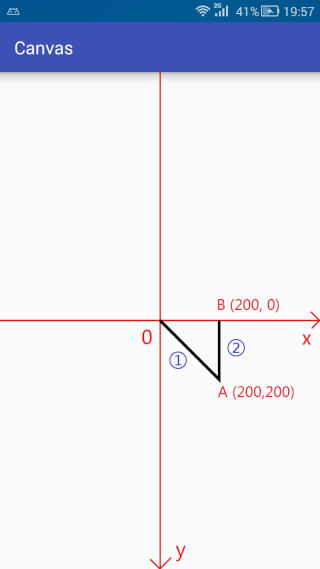#### moveTo 和 setLastPoint：

``````// moveTo
public void moveTo (float x, float y)

// setLastPoint
public void setLastPoint (float dx, float dy)
``````

moveTo 移动下一次操作的起点位置
setLastPoint 设置之前操作的最后一个点位置

``````canvas.translate(mWidth / 2, mHeight / 2);  // 移动坐标系到屏幕中心

Path path = new Path();                     // 创建Path

path.lineTo(200, 200);                      // lineTo

path.moveTo(200,100);                       // moveTo

path.lineTo(200,0);                         // lineTo

canvas.drawPath(path, mPaint);              // 绘制Path
``````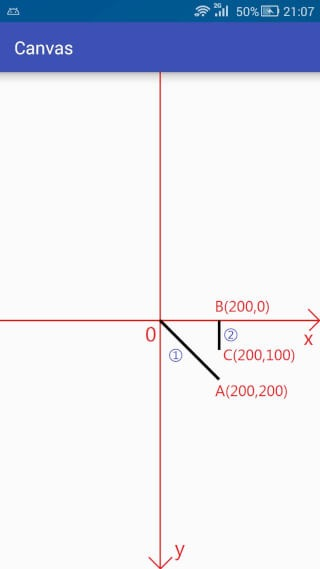moveTo只改变下次操作的起点，在执行完第一次LineTo的时候，本来的默认点位置是A(200,200),但是moveTo将其改变成为了C(200,100),所以在第二次调用lineTo的时候就是连接C(200,100) 到 B(200,0) 之间的直线(用蓝色圈2标注)。

``````canvas.translate(mWidth / 2, mHeight / 2);  // 移动坐标系到屏幕中心

Path path = new Path();                     // 创建Path

path.lineTo(200, 200);                      // lineTo

path.setLastPoint(200,100);                 // setLastPoint

path.lineTo(200,0);                         // lineTo

canvas.drawPath(path, mPaint);              // 绘制Path
``````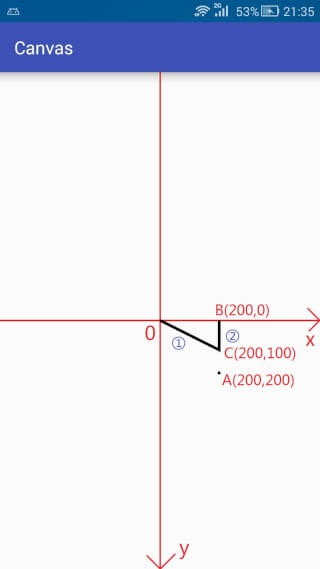setLastPoint是重置上一次操作的最后一个点，在执行完第一次的lineTo的时候，最后一个点是A(200,200),而setLastPoint更改最后一个点为C(200,100),所以在实际执行的时候，第一次的lineTo就不是从原点O到A(200,200)的连线了，而变成了从原点O到C(200,100)之间的连线了。

#### close

``````public void close ()
``````

close方法用于连接当前最后一个点和最初的一个点(如果两个点不重合的话)，最终形成一个封闭的图形。

``````canvas.translate(mWidth / 2, mHeight / 2);  // 移动坐标系到屏幕中心

Path path = new Path();                     // 创建Path

path.lineTo(200, 200);                      // lineTo

path.lineTo(200,0);                         // lineTo

path.close();                               // close

canvas.drawPath(path, mPaint);              // 绘制Path
``````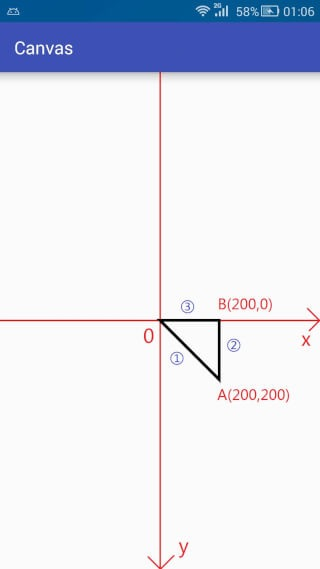#### 第一类(基本形状)

``````// 第一类(基本形状)

// 圆形
// 椭圆
public void addOval (RectF oval, Path.Direction dir)
// 矩形
public void addRect (float left, float top, float right, float bottom, Path.Direction dir)
public void addRect (RectF rect, Path.Direction dir)
// 圆角矩形
public void addRoundRect (RectF rect, float rx, float ry, Path.Direction dir)
``````

Direction的意思是 方向，趋势。 点进去看一下会发现Direction是一个枚举(Enum)类型，里面只有两个枚举常量，如下：

CW clockwise 顺时针
CCW counter-clockwise 逆时针

1 在添加图形时确定闭合顺序(各个点的记录顺序)
2 对图形的渲染结果有影响(是判断图形渲染的重要条件)

``````canvas.translate(mWidth / 2, mHeight / 2);  // 移动坐标系到屏幕中心

Path path = new Path();

canvas.drawPath(path,mPaint);
``````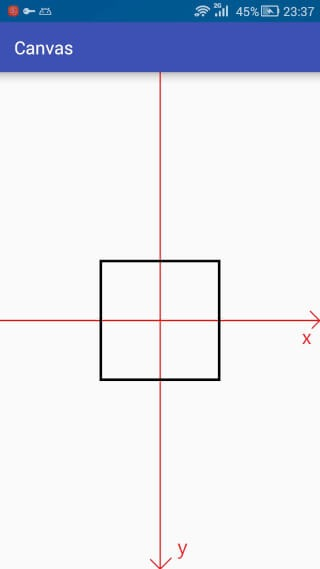(╯°Д°)╯︵ ┻━┻(再TM掀一次) 坑人也不带这样的啊，一毛一样要它干嘛。

``````canvas.translate(mWidth / 2, mHeight / 2);  // 移动坐标系到屏幕中心

Path path = new Path();

path.setLastPoint(-300,300);                // <-- 重置最后一个点的位置

canvas.drawPath(path,mPaint);
``````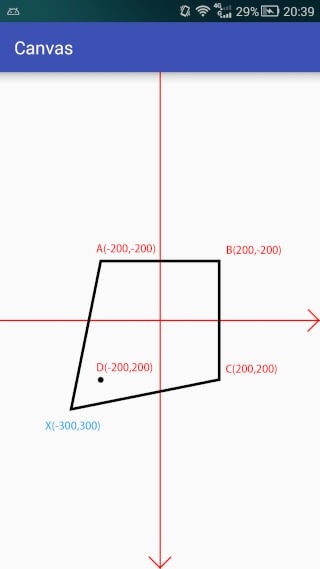``````canvas.translate(mWidth / 2, mHeight / 2);  // 移动坐标系到屏幕中心

Path path = new Path();

path.setLastPoint(-300,300);                // <-- 重置最后一个点的位置

canvas.drawPath(path,mPaint);
``````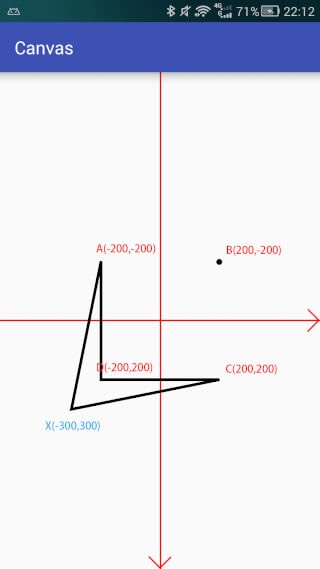#### 第二类(Path)

``````// 第二类(Path)
// path
public void addPath (Path src, float dx, float dy)
public void addPath (Path src, Matrix matrix)
``````

``````canvas.translate(mWidth / 2, mHeight / 2);  // 移动坐标系到屏幕中心
canvas.scale(1,-1);                         // <-- 注意 翻转y坐标轴

Path path = new Path();
Path src = new Path();

mPaint.setColor(Color.BLACK);           // 绘制合并后的路径
canvas.drawPath(path,mPaint);
``````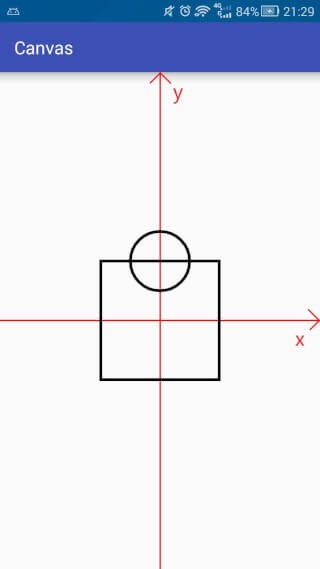``````// 第三类(addArc与arcTo)

public void addArc (RectF oval, float startAngle, float sweepAngle)
// arcTo
public void arcTo (RectF oval, float startAngle, float sweepAngle)
public void arcTo (RectF oval, float startAngle, float sweepAngle, boolean forceMoveTo)
``````

oval 圆弧的外切矩形。
startAngle 开始角度
sweepAngle 扫过角度(-360 <= sweepAngle <360)
forceMoveTo 是否强制使用MoveTo

PS: sweepAngle取值范围是 [-360, 360)，不包括360，当 >= 360 或者 < -360 时将不会绘制任何内容， 对于360，你可以用一个接近的值替代，例如: 359.99。

arcTo 添加一个圆弧到path 添加一个圆弧到path，如果圆弧的起点和上次最后一个坐标点不相同，就连接两个点

forceMoveTo是什么作用呢？

forceMoveTo 含义 等价方法
true 将最后一个点移动到圆弧起点，即不连接最后一个点与圆弧起点 public void addArc (RectF oval, float startAngle, float sweepAngle)
false 不移动，而是连接最后一个点与圆弧起点 public void arcTo (RectF oval, float startAngle, float sweepAngle)

``````canvas.translate(mWidth / 2, mHeight / 2);  // 移动坐标系到屏幕中心
canvas.scale(1,-1);                         // <-- 注意 翻转y坐标轴

Path path = new Path();
path.lineTo(100,100);

RectF oval = new RectF(0,0,300,300);

// path.arcTo(oval,0,270,true);             // <-- 和上面一句作用等价

canvas.drawPath(path,mPaint);
``````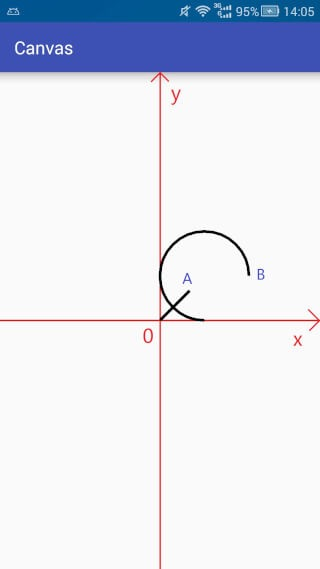``````canvas.translate(mWidth / 2, mHeight / 2);  // 移动坐标系到屏幕中心
canvas.scale(1,-1);                         // <-- 注意 翻转y坐标轴

Path path = new Path();
path.lineTo(100,100);

RectF oval = new RectF(0,0,300,300);

path.arcTo(oval,0,270);
// path.arcTo(oval,0,270,false);             // <-- 和上面一句作用等价

canvas.drawPath(path,mPaint);
``````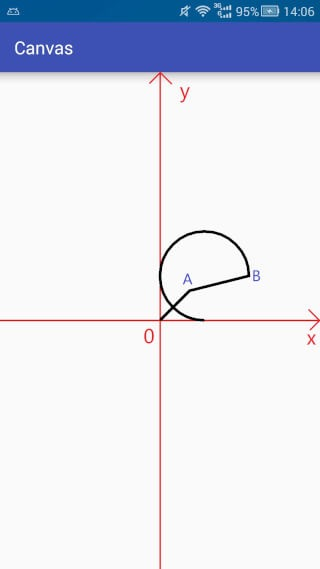### 第3组：isEmpty、 isRect、isConvex、 set 和 offset

#### isEmpty

``````public boolean isEmpty ()
``````

``````Path path = new Path();
Log.e("1",path.isEmpty()+"");

path.lineTo(100,100);
Log.e("2",path.isEmpty()+"");
``````

log输出结果:

``````com.sloop.canvas E/1: true
com.sloop.canvas E/2: false
``````

#### isRect

``````public boolean isRect (RectF rect)
``````

``````path.lineTo(0,400);
path.lineTo(400,400);
path.lineTo(400,0);
path.lineTo(0,0);

RectF rect = new RectF();
boolean b = path.isRect(rect);
Log.e("Rect","isRect:"+b+"| left:"+rect.left+"| top:"+rect.top+"| right:"+rect.right+"| bottom:"+rect.bottom);
``````

log 输出结果:

``````com.sloop.canvas E/Rect: isRect:true| left:0.0| top:0.0| right:400.0| bottom:400.0
``````

#### set

``````public void set (Path src)
``````

``````canvas.translate(mWidth / 2, mHeight / 2);  // 移动坐标系到屏幕中心
canvas.scale(1,-1);                         // <-- 注意 翻转y坐标轴

Path path = new Path();                     // path添加一个矩形

Path src = new Path();                      // src添加一个圆

path.set(src);                              // 大致相当于 path = src;

canvas.drawPath(path,mPaint);
``````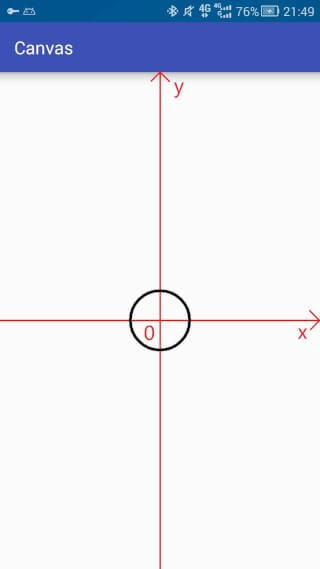#### offset

``````public void offset (float dx, float dy)
public void offset (float dx, float dy, Path dst)
``````

dst状态 效果
dst不为空 将当前path平移后的状态存入dst中，不会影响当前path
dst为空(null) 平移将作用于当前path，相当于第一种方法

``````canvas.translate(mWidth / 2, mHeight / 2);  // 移动坐标系到屏幕中心
canvas.scale(1,-1);                         // <-- 注意 翻转y坐标轴

Path path = new Path();                     // path中添加一个圆形(圆心在坐标原点)

Path dst = new Path();                      // dst中添加一个矩形

path.offset(300,0,dst);                     // 平移

canvas.drawPath(path,mPaint);               // 绘制path

mPaint.setColor(Color.BLUE);                // 更改画笔颜色

canvas.drawPath(dst,mPaint);                // 绘制dst
``````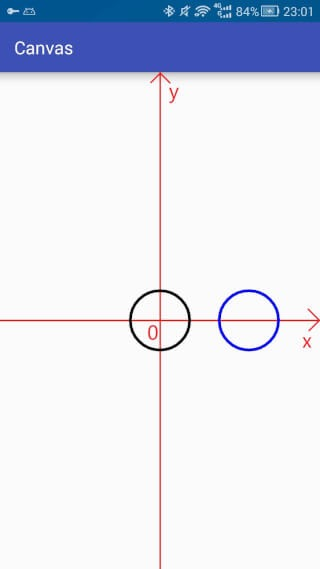# 三.总结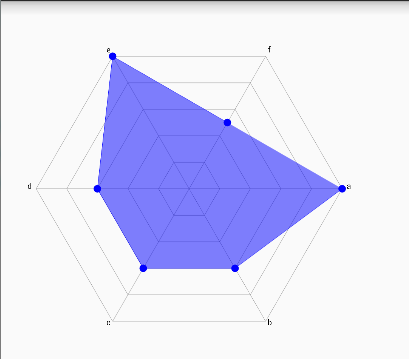(,,• ₃ •,,)

PS: 由于本人水平有限，某些地方可能存在误解或不准确，如果你对此有疑问可以提交Issues进行反馈。

¥ 点击赞助

## 欢迎关注我的微信公众号Course

CustomView